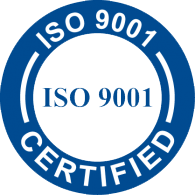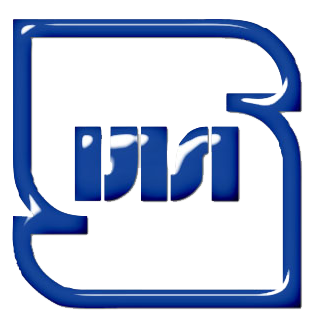Concrete Testing Equipment

# Concrete Testing Equipment

Concrete tensile strength test (Brazilian test)

This test in order to determine the indirect single axis tensile strength of a cylindrical concrete samples which is known as the Brazilian test In fact. A tensile stress is applied on concrete and other stress which the value is three times of the maximum tensile stress apply as Compressive In this case, single axis tensile stress the breakage of tensile will achieve.

 Standard: BS EN 12390-5

Ordering
Code
Description
Sample
Size
Cm
Dimension
Cm
Weight
Approx.
Kg
Material

SMCO-3610

Concrete tensile strength test

Ø10×20

34×19×20

14.5

Iron sheet chrome plating

SMCO-3630

Concrete tensile strength test

Ø15×30

44×25×26

31

Iron sheet chrome plating

Elasticity Modulus and Poisson's ratio test (Young's modulus)

Modulus of elasticity or Young's modulus is a method of measuring the strain rate as a function of stress. The applied stress can be compressive strength or tensile strength of concrete samples, and this represents the slope of the linear part of a stress-strain curves. The elasticity modulus of concrete is a key factor to estimate the deformation of structural elements, as well as a fundamental factor to determine the modular ratio, N, used for designing of structures which is subjected to bending, on the basis of concrete elasticity modulus which is proportional to the square root of compressive strength of ordinary concrete strength the following equation specifies to estimate the elasticity modulus of concrete.

 Standard: ASTM C469

Ordering
Code
Description
Dimension
Cm
Weight
Approx.
Kg

Material

Appurtenance

SMCO-3640

Elasticity Modulus and Poisson's ratio test
for Ø4" Speciment

Ø20×15.5

1.400

Aluminum
CK45
2 Dial Gauges
(5mm travel &
0.002mm divisions)

SMCO-3645

Elasticity Modulus and Poisson's ratio test
for Ø6" Speciment

Ø27×28.5

3.700

Aluminum
CK45
2 Dial Gauges
(5mm travel &
0.002mm divisions)
 ADD: No. 52, 12m ROOZ Alley, 16m BOSTAN St.,         ZARRIN DASHT Industrial Zone, DABAGHCHI St.,         HAFTJOY City, SHAHRYAR-KARAJ Road, IRAN TEL:  +98-21-4689 3980 (for FAX press 4) CEO CELLPHONE: +98-912-245 5845 E-mail: This email address is being protected from spambots. You need JavaScript enabled to view it.